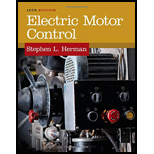# A stator winding of a wound rotor motor contains six poles per phase. If it is connected to a 60 Hz power source, what is the synchronous speed? a. 3600 RPM b. 900 RPM c. 1800 RPM d. 1200 RPMBuyFind

### Electric Motor Control

10th Edition
Herman
Publisher: CENGAGE L
ISBN: 9781133702818BuyFind

### Electric Motor Control

10th Edition
Herman
Publisher: CENGAGE L
ISBN: 9781133702818

#### Solutions

Chapter 37, Problem 1SQ
Textbook Problem

## A stator winding of a wound rotor motor contains six poles per phase. If it is connected to a 60 Hz power source, what is the synchronous speed?a. 3600 RPMb. 900 RPMc. 1800 RPMd. 1200 RPM

Expert Solution
To determine

Choose the correct option to find the synchronous speed of a stator winding of a wound rotor containing six poles per phase.

## Answer to Problem 1SQ

The correct option is d.1200RPM.

### Explanation of Solution

Given data:

The number of poles per phase (p) is 6.

The frequency of the motor (f) is 60Hz.

Formula used:

Write the general expression to find the synchronous speed.

Ns=120fp (1)

Here,

f is the frequency of the motor,

p is the number of poles per phase.

Calculation:

Substitute 60Hz for f, and 6 for p in Equation (1).

Ns=120(60Hz)6=1200RPM

Therefore, the synchronous speed is 1200RPM.

Conclusion:

Thus, the correct option is d.1200RPM.

### Want to see more full solutions like this?

Subscribe now to access step-by-step solutions to millions of textbook problems written by subject matter experts!

### Want to see more full solutions like this?

Subscribe now to access step-by-step solutions to millions of textbook problems written by subject matter experts!

#### Additional Engineering Textbook Solutions

Find more solutions based on key concepts
What is the kernel of the operating system?

Fundamentals of Information Systems

How has technology changed in recent years? Provide of traditional, evolving and emerging technology.

Systems Analysis and Design (Shelly Cashman Series) (MindTap Course List)

What are the three basic operations in cryptography?

Principles of Information Security (MindTap Course List)

What is the inch equivalent of 1 millimeter?

Precision Machining Technology (MindTap Course List)

What is a cost-benefit analysis?

Management Of Information Security

The value of viscosity of a fluid represents a measure of how easily the given fluid can flow. The higher the v...

Engineering Fundamentals: An Introduction to Engineering (MindTap Course List)

List five subtypes of DoS attacks.

Network+ Guide to Networks (MindTap Course List)

Describe CMOS technology and its possible uses.

Enhanced Discovering Computers 2017 (Shelly Cashman Series) (MindTap Course List)

If your motherboard supports ECC DDR3 memory, can you substitute non-ECC DDR3 memory?

A+ Guide to Hardware (Standalone Book) (MindTap Course List)© 2021 bartleby. All Rights Reserved.